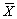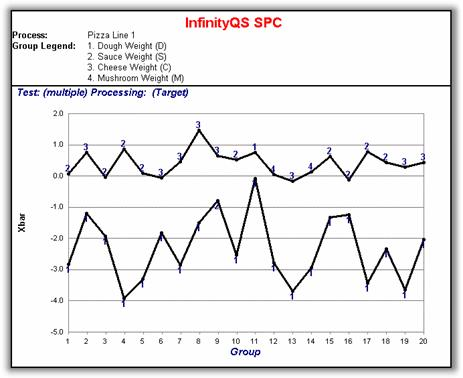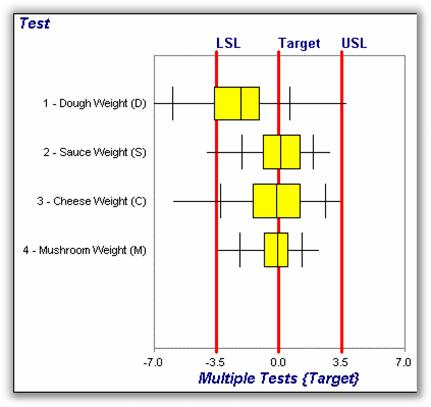# How ProFicient SPC Software Can Track Multiple Frozen Food Ingredients on a Single Control Chart

This application note will show one approach as to how ProFicient SPC Software can be implemented in a frozen food environment. In this pizza example, ingredients are incrementally added and weights are calculated by subtracting off the weights of previous operations. The line is currently producing mushroom pizzas. Figure 1 describes the production flow.

Figure 1 Pizza assembly line.

Ingredient weights are taken from 5 consecutive pizzas, every 30 minutes, at each of the four operations (dough, sauce, cheese and mushroom). Table 1 below contains the values from the first sampling period.

Table 1 Data points from one subgroup. Each subgroup consists of five readings for each ingredient.

## The Group Control Chart

Using traditional SPC methods, one could set up a separateand R control chart for each characteristic. However, InfinityQS SPC has powerful, innovative Group charts that allow users to extract far more meaningful information from the data. Rather than four control charts, one can use a single Group control chart to evaluate all operations from the production line. Group control charts combine multiple process streams onto a single chart. In order to combine all ingredients on the same chart, the data must first be coded to fit a common scale. Data is coded by subtracting each ingredient target weight from the subgroup average. This coding is sometimes called DFT (deviation from target). Once DFT values are calculated, the two characteristics furthest away, low and high, from their respective target values are plotted.

Table 2 Deviation from target calculations. Target values come from the specification limit record.

## Deviation from Target—DTF Data

In Table 2, the dough weight (-3.84) and the sauce weight (0.06) are the lowest and highest values. With DFT data, all four ingredient weights can be plotted on the same Group chart. Group charts only plot the lowest and highest values within a grouping of data. The high and low plot points are labeled with a number that corresponds to a characteristic.Figure 2 Group Control Chart.

In Figure 2, the Max line shows a random pattern of 2, 3 and 4. The majority of plot points on the Min line are 1 - dough weight.

On one group control chart, all four ingredients can be monitored. Traditional control charts just were not designed to provide this level of real-time information.

## The Box Plot

Another graphical tool to consider is the Box Plot. Like a histogram, the Box Plot illustrates a data's distribution (Figure 3).Figure 3 Box Plot interpretation.

In Figure 4, the Box Plot clearly shows that Dough Weight is furthest off target and exhibits the greatest amount of variation. Mushroom Weight exhibits the least amount of variation.

Adding the Group chart and Box Plot to your toolbox can significantly improve the value of your company's Statistical Process Control (SPC) program.Figure 4 Box Plot illustrating all four ingredients plotted as deviation from target.

Need help?
​For further information contact GetInTouch@infinityqs.com
Toll Free: 1.800.772.7978​

### Take the first step from quality to excellence

Take the Next Steps